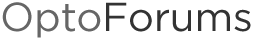# Simple Sort for Hour Meters

I needed a simple sorting routine and everything I looked at got my eyes glazing over. So I came up with this.

I needed to start fans with lowest hours, so the fan pTable is loading the Fan pointer with the first index from the hour meter table and then comparing that hour meter to all other hour meters in the table. Each time it is lower or equal, it adds one to the SortOrder_nTable at the current index of the Sort Index. The HmIndex is used to iterate through all 10 elements of the Hm table comparing the same element from the same table. The next iteration is with element 2 of the Hm table and each complet iteration sum (now in the sort table) is then subtracted from the total number of indexes and put back in that same sort table index. This is the sorted index ranking for that index. When all the iterations are done, the sort table has a collection of rankings that are related to the hour meter table index for the sorted order of each hour meter element in the hour meter table.
This could be taken further to put these indexes in order from 1-10 in another table if needed. Also I suspect one could use this to sort alpha by using ascii code to sort the characters.

1 Like

Cool, looks like an insertion sort - which is fast for small lists. I like that the Sort Order table can be used as an index reference for multiple tables. None of the sorting routines on the forum do that, I like it.

I don’t think you need to zero out (sequentially initialize?) the Sort Order table each time - only needs done one time on power up. This will improve the sorting performance since the list will already be mostly sorted. Probably no big deal for what you are doing though.

Any thoughts on making this into a subroutine?

No I had not considered a subroutine, but it’s a good idea. I’m not sure what you mean by insertion sort, but maybe I can give a better idea of how it works. Essentially, it’s just adding together the number of times the compare value <= to each table value (or in this case the hour meters for all fans), then subtracting from the total number of values being compared to, then adding one and storing that in the sort table. That in essence is the ranking value of the compare value. This value is stored in the same index as the compare value is stored in in the hour meter table. Therefore, I have created a ranking (or index number) for that hour meter. Actually there is no hour meter table in this case, it is just using the index number to point to the actual hour meter variable.
The reason I clear the sort table is that these values are added in, so if not cleared, it will add to whatever is in there. On the other hand, this could also be done one at a time as the table is sorted.
I thought your quick sort looked really sophisticated but wasn’t real sure what you were doing there, and since I was fairly confident that this would work, I just kept playing with it until I got it working. I finally got it close and then realized all the values were exactly one less than what they should be so I added one to the value. I also figured out that it has to be <= not just < and therefore if you sort a list that is all the same value, it will all be index 1.

Okay I misunderstood - I saw a pattern in your code that made me think it was insertion sort, but what you are really doing is ranking the fans by lowest hours to highest hours. You then go through the CO_SortOrder_nTable and find the one with 1 in it the ranking and that is the next fan to start after this?

FWIW, while figuring out how your code works, I made some changes that IMO simplify it somewhat - biggest thing is only dealing with the start and end index of 1 and 10 and not having 0s and 11s thrown in - does the same thing as what you already wrote, but will be easier to edit in the future if you need to work with 11 fans:

CO_SortIndex = 0; //set the flag to run test

if(CO_SortIndex == 0)then

``````//one out table
for CO_SortIndex = 1 to 10 step 1
CO_SortOrder_nTable[CO_SortIndex] = 1;
next

//North sort order
CO_SortIndex = 1;
CO_HmIndex = 10;
repeat
CO_Compare_ptr = Fan_HourMeter_pTable[CO_SortIndex];

repeat
CO_FanHourMeter_ptr = Fan_HourMeter_pTable[CO_HmIndex];

//compare
if(*CO_Compare_ptr > *CO_FanHourMeter_ptr)then
CO_SortOrder_nTable[CO_SortIndex] = 1 + CO_SortOrder_nTable[CO_SortIndex];
endif

DecrementVariable(CO_HmIndex);

until CO_HmIndex < 1;

IncrementVariable(CO_SortIndex);
CO_HmIndex = 10;
until CO_SortIndex > 10;
``````

endifI’m not sure what I was doing there anymore either. To get that working, I had notes with arrows and boxes of numbers all over the place. The lack of recursion in Optoscript doesn’t help make these tasks any easier either.

You must be an oldie but goodie like me…CRS.

Check out my latest issue, SoftPac Speed.

CRS? Not sure what that is…

1 Like

Can’t remember &^%\$…

I knew that… just couldn’t remember